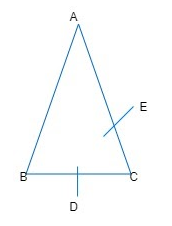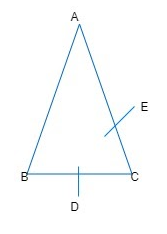# Three charges each have $+q$ charge, are placed at the corner of an isosceles triangle ABC of sides BC and AC, 2a.D and E are the mid points of BC and CA. The work done in taking a charge Q from D to E is:(A) $\dfrac{eqQ}{8\pi {{\in }_{0}}a}$ (B) $\dfrac{qQ}{4\pi {{\in }_{0}}a}$ (C) Zero(D) $\dfrac{3qQ}{4\pi {{\in }_{0}}a}$Verified
183.6k+ views
Hint: To find out the work done is taking charge Q from one point to another, we will find electrostatic potential at those points using
$V=\dfrac{q}{4\pi {{\in }_{0}}r}$
$V$ is the potential generated between the charges
$q$ is the charge due to which our test charge is affected
r is the distance between the charges.

Complete step by step solutionHere,
$AC=BC=2a$
As D and E are midpoints of BC and AC (given).
$\therefore$ $AE=EC=a$
And,
$BD=DC=a$
In $\Delta ADC,$
\begin{align} & {{(AD)}^{2}}={{(AC)}^{2}}-{{(DC)}^{2}} \\ & ={{(2a)}^{2}}-{{(a)}^{2}}={{(4a)}^{2}}-{{(a)}^{2}}={{(a)}^{2}} \\ & AD=a\sqrt{3} \\ \end{align}
Similarly, potential at points D due to the given charge distribution is
${{V}_{D}}=\dfrac{1}{4\pi {{\in }_{0}}}[\dfrac{q}{BD}+\dfrac{q}{DC}+\dfrac{q}{AD}]$
\begin{align} & =\dfrac{q}{4\pi {{\in }_{0}}}\left[ \dfrac{1}{a}+\dfrac{1}{a}+\dfrac{1}{\sqrt{3}a} \right] \\ & =\dfrac{q}{4\pi {{\in }_{0}}a}\left[ 2+\dfrac{1}{\sqrt{3}} \right] \\ \end{align} ...........................(1)
Potential at point E due to given charge configuration is
${{V}_{E}}=\dfrac{1}{4\pi {{\in }_{0}}}\left[ \dfrac{1}{q}+\dfrac{1}{q}+\dfrac{1}{a\sqrt{3}} \right]$
$=\dfrac{q}{4\pi {{\in }_{0}}a}\left[ 2+\dfrac{1}{\sqrt{3}} \right]$ ...........................(2)
From (1) and (2), it is clear that
The work done in taking a charge Q from D to E is
$W=Q({{V}_{E}}-{{V}_{D}})=0$ $(\because {{V}_{D}}={{V}_{E}})$
Therefore, option (C) is correct.

Note
Electric potential due to single charge is spherically symmetric. It should be clearly borne in mind that due to single charge,
$F\propto \dfrac{1}{{{r}^{2}}}$ ; $E\propto \dfrac{1}{{{r}^{2}}}$ but $V\propto \dfrac{1}{r}$ ,
where r is the distance from the charge.